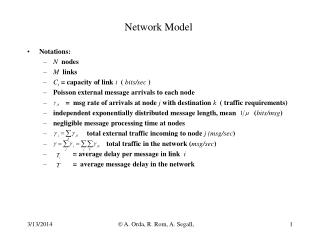DownloadDownload PresentationNetwork Model

# Network Model

Télécharger la présentation## Network Model

- - - - - - - - - - - - - - - - - - - - - - - - - - - E N D - - - - - - - - - - - - - - - - - - - - - - - - - - -
##### Presentation Transcript

1. Network Model • Notations: • N nodes • M links • Ci = capacity of link i ( bits/sec ) • Poisson external message arrivals to each node • = msg rate of arrivals at node j with destination k ( traffic requirements) • independent exponentially distributed message length, mean (bits/msg) • negligible message processing time at nodes • total external traffic incoming to node j (msg/sec) • total traffic in the network (msg/sec) • = average delay per message in link i • = average message delay in the network  A. Orda, R. Rom, A. Segall,

2. Delay calculations • Assumption: non-splitting fixed routing • Notations: • route from node j to node k • link-route indicator: • Total traffic on link i namely traffic from j to k is included if the path includes link i • Zjk= Average Delay on path from j to k namely delay on link i is included only if it is part of the path from j to k  A. Orda, R. Rom, A. Segall,

3. example • Path from A to L • by links • by nodes • Examples: Therefore: Average Network Delay in a general network  A. Orda, R. Rom, A. Segall,

4. Calculation of average delay Tifor a single (separate) link • Messages arrive to the link have exponential length with parameter , namely therefore service time is random. If the bit rate (capacity) of the link is Ci bits/sec, then transmission time is: therefore transmission time is exponentially distributed with parameter and Calculation of network average delay T • Question: is the quantity Ti calculated above the same as Tiused earlier? • Answer: No, since normally the service times at consecutive nodes are not independent and not independent of the arrival process. This is because message length remains the same. • Kleinrock’s assumption: service times at different nodes are independent, good approximation because there are many sources and many destinations. • This allows us to calculate average network delay T where is the flow in bits/sec on link i.  A. Orda, R. Rom, A. Segall,

5. Design Problems • Capacity assignment: Given set of nodes and links, flows fi or , budget D. Find link capacities to minimize network average delay T. • Routing ( flow assignment ): Given network and link capacities. Find flows to minimize average delay T. • Capacity and Flow assignment: Given budget D. Find capacities Ci and flows to minimize average delay T.  A. Orda, R. Rom, A. Segall,

6. Capacity Assignment • Given: • fi = flow on link i • network topology • link cost, proportional to speed • total budget D • Find link capacities Ci such that delay T will be minimized • Optimization problem: • Solution: Lagrangian:  A. Orda, R. Rom, A. Segall,

7. Capacity Assignment Denote the extra-budget: • Conclusions: • Capacity of link l should be at least (stability) • The extra-budget De is divided by normalizing it to di and using square root assignment • thus T is inversely proportional to De ( larger budget, better performance).  A. Orda, R. Rom, A. Segall,